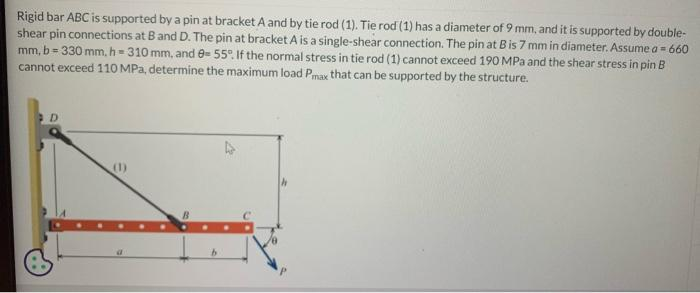Home / Expert Answers / Civil Engineering / rigid-bar-abc-is-supported-by-a-pin-at-bracket-a-and-by-tie-rod-1-tie-rod-1-has-a-diamet-pa258

# (Solved): Rigid bar ABC is supported by a pin at bracket $$A$$ and by tie rod (1). Tie rod (1) has a diamet ...Rigid bar ABC is supported by a pin at bracket $$A$$ and by tie rod (1). Tie rod (1) has a diameter of $$9 \mathrm{~mm}$$, and it is supported by doubleshear pin connections at $$B$$ and $$D$$. The pin at bracket $$A$$ is a single-shear connection. The pin at $$B$$ is $$7 \mathrm{~mm}$$ in diameter. Assume $$a=660$$ $$\mathrm{mm}, \mathrm{b}=330 \mathrm{~mm}, \mathrm{~h}=310 \mathrm{~mm}$$, and $$\theta=55^{\circ}$$. If the normal stress in tie rod (1) cannot exceed $$190 \mathrm{MPa}$$ and the shear stress in pin $$B$$ cannot exceed $$110 \mathrm{MPa}$$, determine the maximum load $$P_{\max }$$ that can be supported by the structure.

We have an Answer from Expert